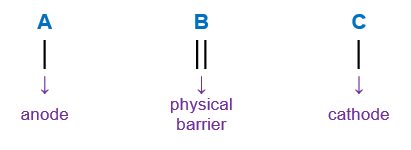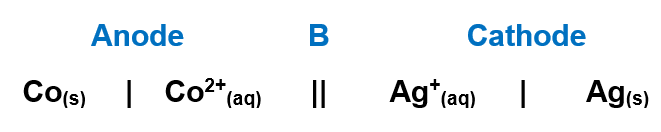# Problem: For the following electrochemical cell Co(s)|Co2+(aq, 0.0155 M)||Ag+(aq, 2.50 M)|Ag(s) write the net cell equation. Phases are optional. Do not include the concentrations. Calculate the following values at 25.0 °C using standard potentials as needed. E°cell = ____ V                                  ΔG°rxn = ____ kJ/molE°cell = ____ V                                  ΔG°rxn = ____ kJ/mol

###### FREE Expert Solution

Step 1: Balanced equation and the number of electrons

When writing a cell notation, we use the following format – “as easy as ABCRecall the mnemonics LEO GER.

Lose               Gain

Electron         Electrons

Oxidation       Reduction

lose electrons → oxidation → anode

gain electrons → reduction → cathodeWe’re going to write the overall balanced reaction equation using the following sub-steps:

Sub-step 1: Write the anode reaction.

Sub-step 2: Write the cathode reaction.

Sub-step 3: Balanced anode and cathode reactions.

Sub-step 4: Get the overall reaction.###### Problem Details

For the following electrochemical cell

Co(s)|Co2+(aq, 0.0155 M)||Ag+(aq, 2.50 M)|Ag(s)

write the net cell equation. Phases are optional. Do not include the concentrations.

Calculate the following values at 25.0 °C using standard potentials as needed.

cell = ____ V                                  ΔG°rxn = ____ kJ/mol

cell = ____ V                                  ΔG°rxn = ____ kJ/mol